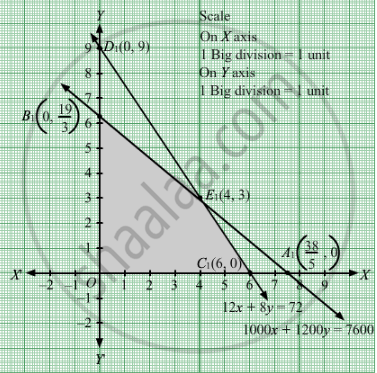# A Factory Owner Purchases Two Types of Machines, a and B, for His Factory. the Requirements and Limitations for the Machines Are as Follows: - Mathematics

Sum

A factory owner purchases two types of machines, A and B, for his factory. The requirements and limitations for the machines are as follows:

 Area occupied by themachine Labour force for eachmachine Daily output inunits Machine AMachine B 1000 sq. m1200 sq. m 12 men8 men 6040

He has an area of 7600 sq. m available and 72 skilled men who can operate the machines.
How many machines of each type should he buy to maximize the daily output?

#### Solution

Let machines of type A and y machines of type B were purchased.
Number of machines cannot be negative.
Therefore,

$x, y \geq 0$

We are given,

 Area occupied by themachine Labour force for eachmachine Daily output inunits Machine AMachine B 1000 sq. m1200 sq. m 12 men8 men 6040

The area of 7600 sq m is available and there are 72 skilled men available to operate the machines.
Therefore, the constraints are

$1000x + 1200y \leq 7600$
$\text{ and } 12x + 8y \leq 72$

Total daily output = Z =  $60x + 40y$\

which is to be maximised.

Thus, the mathematical formulat​ion of the given linear programming problem is

Max Z =  $60x + 40y$

subject to

$1000x + 1200y \leq 7600$
$12x + 8y \leq 72$

$x, y \geq 0$

First we will convert inequations into equations as follows :
1000x + 1200y = 7600, 12x + 8y = 72, x = 0 and y = 0
Region represented by 1000x + 1200y ≤ 7600:
The line 1000x + 1200y = 7600 meets the coordinate axes at $A_1 \left( \frac{38}{5}, 0 \right)$ and $B_1 \left( 0, \frac{19}{3} \right)$ respectively. By joining these points we obtain the line
1000x + 1200y = 7600. Clearly (0,0) satisfies the 1000x + 1200y = 7600. So, the region which contains the origin represents the solution set of the inequation 1000x + 1200y ≤ 7600.
Region represented by 12x + 8y ≤ 72:
The line 12x + 8y = 72 meets the coordinate axes at C1(6, 0) and D1(0, 9) respectively. By joining these points we obtain the line 12x + 8y = 72 .Clearly (0,0) satisfies the inequation 12x + 8y ≤ 72. So,the region which contains the origin represents the solution set of the inequation 12x + 8y ≤ 72.
Region represented by x ≥ 0 and y ≥ 0:
Since, every point in the first quadrant satisfies these inequations. So, the first quadrant is the region represented by the inequations x ≥ 0, and ≥ 0.
The feasible region determined by the system of constraints 1000x + 1200y ≤ 7600, 12x+ 8y ≤ 72, x ≥ 0, and y ≥ 0 are as follows.The corner points are O(0, 0)

$B_1 \left( 0, \frac{19}{3} \right)$ , E1(4, 3), C1(6, 0)
The values of Z at these corner points are as follows

 Corner point Z= 60x + 40y O 0 B1 253.3 E1 360 C1 360

The maximum value of Z is 360 which is attained at E1(4, 3) and C1(6, 0).

Thus, the maximum output is Rs 360 obtained when 4 units of type A and 3 units of type B or 6 units of type A are manufactured.

Concept: Graphical Method of Solving Linear Programming Problems
Is there an error in this question or solution?

#### APPEARS IN

RD Sharma Class 12 Maths
Chapter 30 Linear programming
Exercise 30.4 | Q 8 | Page 51